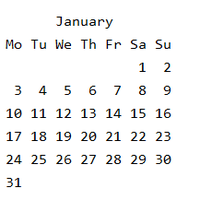# Calculate current week number in JavaScript

• Last Updated : 24 May, 2022

The week number indicates the number of weeks that have been completed in the current year.

Example:

```The week number of january 1  = week 1
The week number of january 30 = week 4```

Explanation: From the below calendar we can see that Jan 1 is in week 1 and Jan 30 in week 5In the process of calculating the current week number, we will the following numbers.

getFullYear(): getFullYear() returns the full year (4 digits) of a date.

Syntax:

`Date.getFullYear()`

getDay():The getDay() method returns the day of the week (0 to 6) of a date.

Syntax:

`Date.getDay()`

Approach: Initialize the current date to a variable using a new Date() which by default returns the current date. Initialize the starting date of the current year ( i.e. Jan 1) to startDate. Calculate the difference between the two dates in days by subtracting startDate from currentDate.

• This returns the difference between dates in milliseconds.
• now dividing the result by total milliseconds in a day gives a difference between dates in days.

Add the number of days to the current weekday using getDay() and divide it by 7. We will get the current week’s number.

Example: Below code will illustrate the above example:

## Javascript

 ``

Output:

```Week number of Mon Feb 14 2022 08:15:31
GMT+0530 (India Standard Time) is : 7```
My Personal Notes arrow_drop_up
Recommended Articles
Page :